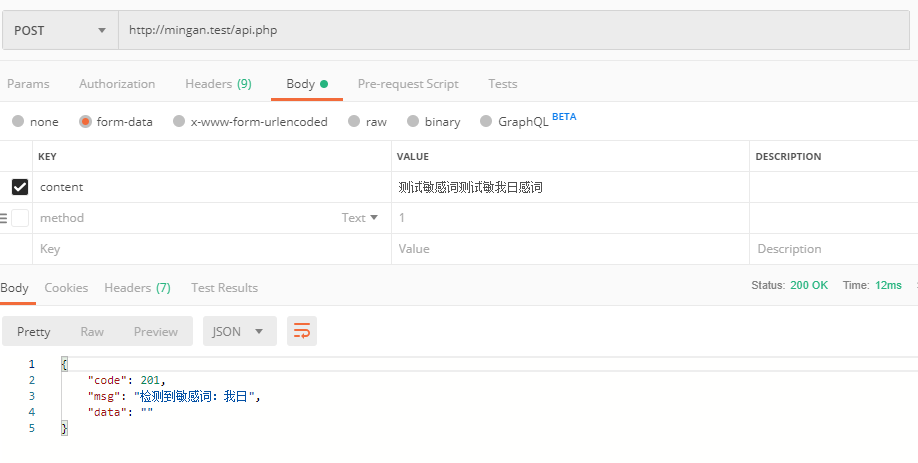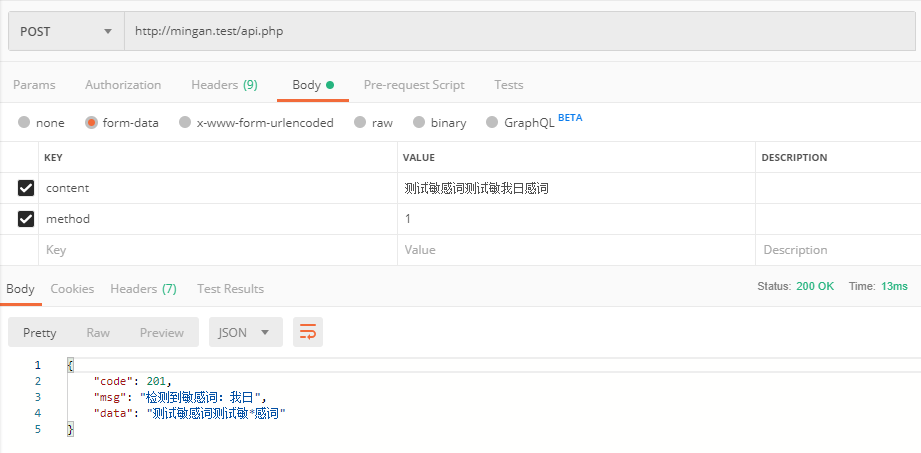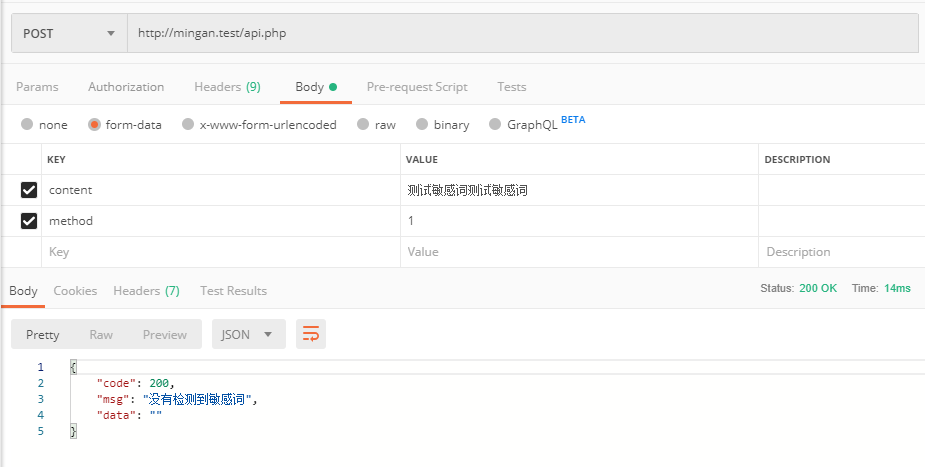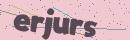# 使用原生PHP写一个敏感词检测过滤接口

```我日

```<?php

\$content = isset(\$_POST['content']) ? \$_POST['content'] : null;
if(!\$content){
return_json(301, '请提交需要检测的内容');
}

if(\$res = check_content(\$content)){
if(isset(\$_POST['method']) && \$_POST['method'] == 1){
//过滤敏感词
\$content = str_replace(\$res, '*', \$content);
return_json(201, '检测到敏感词：' . \$res, \$content);
}
return_json(201, '检测到敏感词：' . \$res);
}
return_json(200, '没有检测到敏感词');

function check_content(\$content)
{
\$file = 'words.txt';
//上面这个就是词库文件路径
\$sensitive_array = array_map('rtrim', file(\$file));
foreach (\$sensitive_array as \$key => \$value) {
if (\$value == ''){
continue;
}
if (mb_strpos(\$content, \$value) != false){
return \$value;
//return true;
}
}
return false;
}

function return_json(\$code, \$msg, \$data = ''){
exit(json_encode([
'code' => \$code,
'msg' => \$msg,
'data' => \$data,
]));
}
?>```

msg => 返回提示 + 敏感词, 无敏感词则返回提示

data => 当接口请求时携带 method 参数且值为 1 时，返回过滤好的内容。(无敏感内容或不携带 method 参数时，该参数返回的结果均为空)